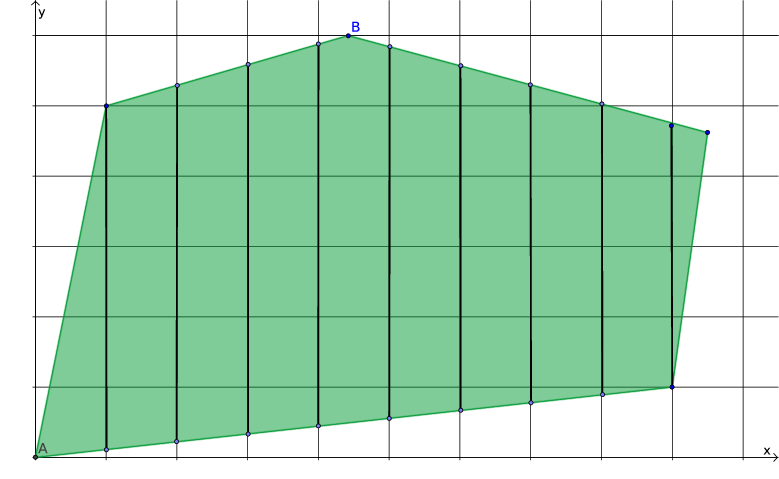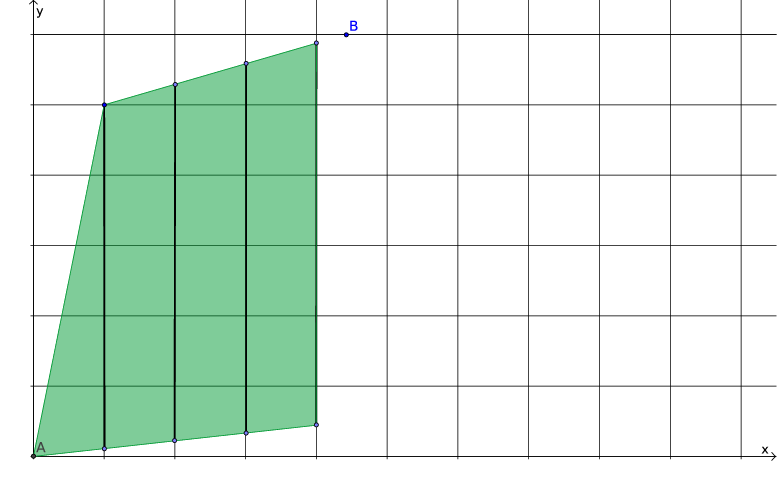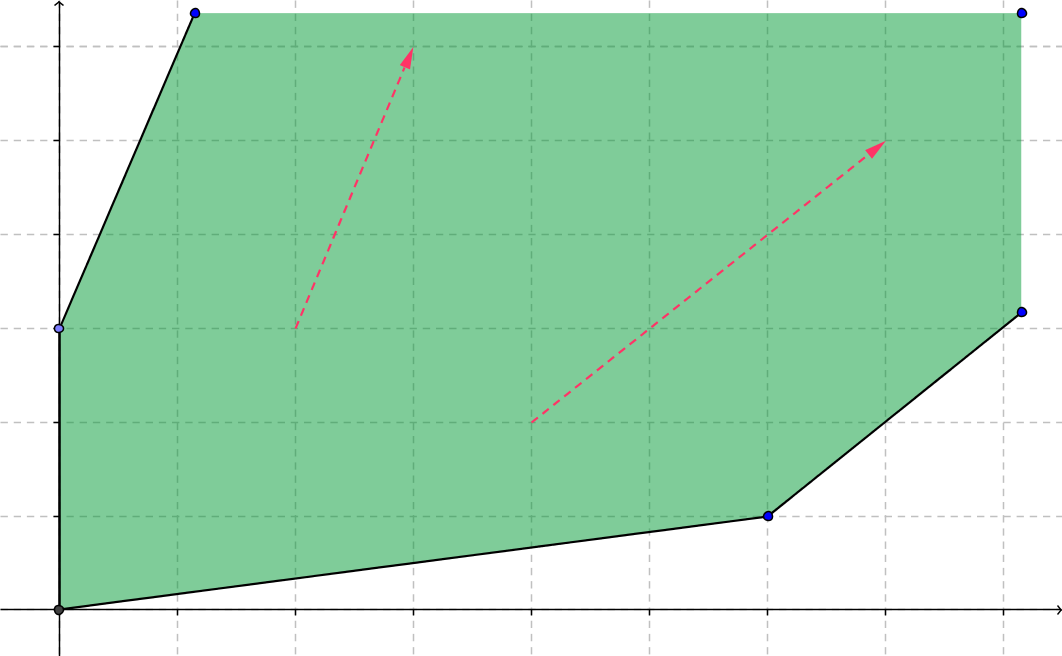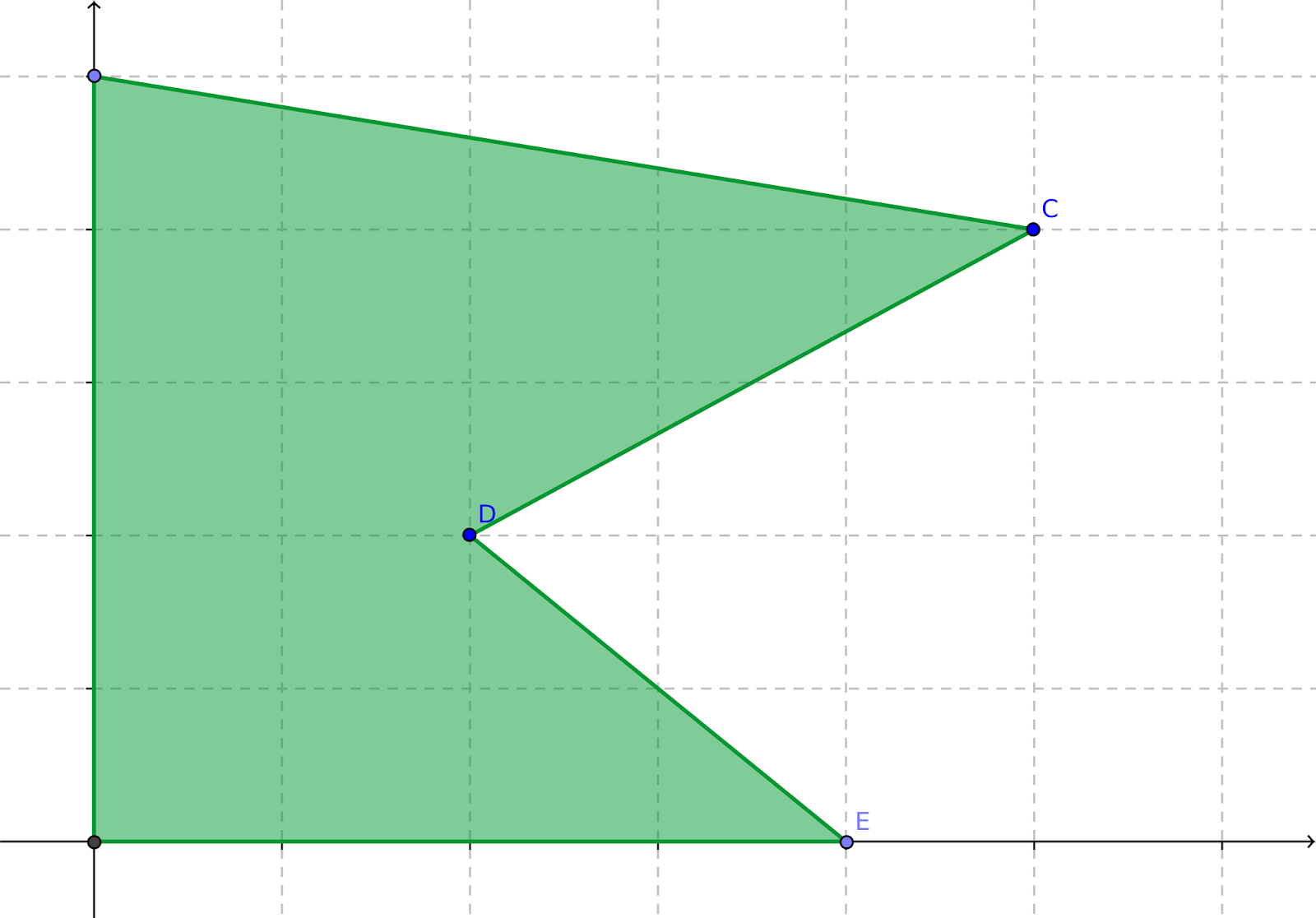## Monday, February 23, 2015

### Partitioning with Binary Variables

Armed with the contents of my last two posts ("The Geometry of a Linear Program", "Branching Partitions the Feasible Region"), I think I'm ready to get to the question that motivated all this. Let me quickly enumerate a few key take-aways from those posts:
• The branch and bound method for solving an integer linear program or mixed integer linear program uses a search tree.
• At each node of the search tree, a linear program (LP) that relaxes some or all of the integrality restrictions (while adding some new restrictions based on earlier branching) is solved. (As an aside, this hold for mixed integer quadratic programs, with the change that the node relaxation will likely be a quadratic program rather than a linear program.)
• LPs have closed and convex feasible regions.
• The act of branching (separating a node into two child nodes) partitions the feasible region for the problem at the parent node into two smaller feasible regions.
Let's move on now. A common use for binary decision variables -- I suspect the most common use -- is to partition the feasible region of a problem into two or more disjoint subregions, corresponding to mutually exclusive scenarios. Consider the example in Figure 1, a mixed integer linear program (MILP) in which $x$ is integer-valued and $y$ is continuous. The green polytope is the LP relaxation; the vertical bars are the actual feasible region of the MILP.Figure 1: Feasible region
Now suppose that there is something going on in the problem that depends on whether $x$ is 3 or less, 4-6, or 7 or more. For instance, if $x$ represents the number of workers deployed on some project, we might need a supervisor for every three workers. We need to partition into those three cases.

A general approach for partitioning a feasible region is to introduce one binary variable for each subregion/scenario, and constrain those variables to add up to 1 (so that exactly one scenario is selected). In our example, we add $z_i \in \{0,1\}$ for $i \in \{1,2,3\}$ along with the constraints\begin{eqnarray*} z_{1}+z_{2}+z_{3} & = & 1\\ x & \le & 3z_{1}+9(1-z_{1})\\ x & \le & 6z_{2}+9(1-z_{2})\\ x & \ge & 4z_{2}\\ x & \ge & 7z_{3}. \end{eqnarray*}Left to the reader as an exercise: confirm that if $z_1=1$ then $0\le x \le 3$, if $z_2=1$ then $4\le x\le 6$, and if $z_3=1$ then $7\le x\le 9$. [Update: See Rob Pratt's comment below for a tighter formulation.]

As a side note, before proceeding further, suppose that what we really want is to ensure that either $x\le 3$ or $x\ge 7$. (Don't ask why; just play along.) We do that the same way I just described, but then also constrain $z_2 = 0$. So the technique for partitioning a feasible region also works for omitting a chunk of a feasible region.

One limitation of this approach is that the subregions must be definable using linear constraints, assuming that you want your problem to remain a MILP. Another (and this is really what motivated me to do this post) is that the partitioning really takes place during the branching process. The algebra splits our feasible region into three disjoint chunks so long as $z_1,z_2,z_3$ are integer, but when we are solving node relaxations some or all of the $z$ variables will be relaxed to continuous.

Let's stick with our three-way split, while also fixing $z_2=0$, so that we force either $x\le 3$ or $x\ge 7$. The conceptual feasible region looks like Figure 2. Again, the actual feasible region for the MILP consists of two clusters of vertical line segments; the shaded polytopes are the relaxations of each scenario.Figure 2: Two scenarios
At some node in the search tree, $z_1$ will be fixed at 1 (and $z_2$ and $z_3$ at 0), and the feasible region of the node relaxation will be the polytope on the left side of Figure 2. At some other node, $z_3$ will be fixed at 1 ($z_1$ and $z_2$ at 0), and the feasible region of the node relaxation will be the polytope on the right side of Figure 2.

What about before any of the $z$ variables are fixed (for instance, at the root node)? Since $z_1$ and $z_3$ can take fractional values, the relaxation at the root node (shown in Figure 3) is the same as what it was before we introduced the $z$ variables.Figure 3: Root node relaxation
To see this, consider what happens if we let $(z_1, z_2, z_3) = (0.5, 0, 0.5)$. The constraints on $x$ become\begin{eqnarray*} x & \le & 3(0.5)+9(1-0.5) = 6\\ x & \le & 6(0)+9(1-0) = 9\\ x & \ge & 4(0) = 0\\ x & \ge & 7(0.5) = 3.5. \end{eqnarray*}Together with the original constraints that defined the sides of the polytope in Figure 1, this gives us the portion of the excluded region where $3.5 \le x \le 6$ (which is most of the pink region). For $x$ between 3 and 3.5 or between 6 and 7, we just need to adjust the $z_1, z_3$ split.

The key takeaway here is that the split we see in Figure 2 actually takes effect during branching, not at the root node (and not at nodes below the root but above the point where the solver branches on one of the $z$ variables). The approach of using binary variables to partition feasible regions is valid because the final solution will obey the integrality restrictions (and thus will belong to one of the disjoint scenarios). Along the road to the final solution, though, we cannot count on the partitioning holding true (and, in the case of excluded regions, we cannot count on the unwanted portion actually be excluded yet).

## Saturday, February 21, 2015

### Branching Partitions the Feasible Region

Yesterday's post got me started on the subject of the geometry of a linear program (LP). Today I want to review another well-known geometric aspect, this time of integer linear programs (ILPs) and mixed integer linear programs (MILPs), that perhaps slips some people's minds when they are wrestling with their models. Most solvers use some variant of the branch and bound (or, more generally, branch and cut) algorithm.

The point I want to make is in the title of this post: branching (more precisely, separating a node in the branch and bound search tree into child nodes) equates to partitioning the feasible region into disjoint chunks. The interpretation of "feasible region" in that last sentence is a bit tricky. On the one hand, the region being partitioned continues to relax the integrality restriction on at least some of the integer variables, adding points not belonging to the original feasible region. On the other hand, outside of the root node, every node problem restricts variables to a subset of the original feasible region. The problem solved at each node is a linear program (LP), sometimes called the node LP. The feasible region of the node LP at any node other than the root is neither a subset nor a superset of the original feasible region; it's a bit of both.

To clarify that (I hope), consider the two variable MILP illustrated in Figure 1, in which $x$ is a general integer variable and $y$ is a continuous variable.Figure 1: MILP feasible region

The feasible region consists of a bunch of disjoint line segments (integer abscissas $x$, arbitrary ordinates $y$), along with an isolated point (labeled A). If we relax the integrality restriction on $x$, we get the convex polytope shown in Figure 2, which is the feasible region of the LP at the root node.Figure 2: LP relaxation

Now suppose that we are looking for the point that maximizes $y$. The optimal solution to the root LP is labeled B in Figure 2. Let's say that $B=(x_0, y_0)$. Note that the value $x_0$ of $x$ at B is not integral.

One possible way to partition the root node is to round the value of $x$ at B up in one child node and down in the other. In effect, this adds the new constraint (cut) $x\le \left\lfloor x_{0}\right\rfloor$ to one child node (call it the left child), and the cut $x \ge \left\lceil x_{0}\right\rceil$ to the other (right) child. Figures 3 and 4 show the child nodes.Figure 3: Left childFigure 4: Right child
I left point B in both figures as a point of reference, but it does not belong to either feasible region.

In this particular (rather trivial) example, the maximum value of $y$ in both children occurs at an integer-feasible point (a corner where $x$ happens to take an integer value), so there will be no further branching. More generally, the algorithm might choose to split either child into either smaller chunks, etc.

One of the questions I saw not long ago asked why a particular solver would branch twice on the same variable. If that variable were binary, it would not make sense. If $z$ is a binary variable that takes a fractional value (say $z = 0.234$) in the solution of a node LP, the cuts $z \le \left\lfloor 0.234\right\rfloor$ and $z \ge \left\lceil 0.234\right\rceil$ equate to $z=0$ and $z=1$, and there is no way to further reduce the domain of $z$. For a general integer variable, such as $x$ in the illustration above, there may be reason to further subdivide the domain of $x$.

My apologies if this all seems quite basic; it is building up to the next post, where I hope to answer a not entirely trivial question.

## Friday, February 20, 2015

### The Geometry of a Linear Program

I frequently see questions on forums, in blog comments, or in my in-box that suggest the person asking the question is either unfamiliar with the geometry of a linear program, unsure of the significance of it, or just not connecting their question to the geometry. This post is just the starting point for addressing some of those questions. (I dislike reading or writing long posts, so I'm "chunking" my answer, and this is the first chunk.)

Ideally, the feasible region of a linear program (henceforth "LP") is a convex polytope, a geometric object that is convex, closed, bounded, and has a finite number of flat sides (intersecting at vertices or extreme points). Figure 1 shows a two dimensional example. The shaded area is the feasible region; the dots are the vertices.Figure 1: Polytope

Being closed and bounded (and therefore, in finite dimensions, compact) ensures, via the extreme value theorem, that any continuous objective function attains its maximum and minimum over the feasible region at one or more points. Of course, the objective function of a linear or quadratic program is continuous. For a linear objective function, we can be more specific: the optimum will be attained at one or more extreme points. So we only need to check the vertices, and this in essence is what the famed simplex algorithm does.

To see why that is important, consider the following candidate for world's most trivial LP:$\begin{array}{lrcl} \textrm{minimize} & x\\ \textrm{subject to} & x & \ge & 0. \end{array}$ Its solution is, shockingly, $x=0$. Now suppose we try to use a strict inequality in the lone constraint:$\begin{array}{lrcl} \textrm{minimize} & x\\ \textrm{subject to} & x & \gt & 0. \end{array}$The lower bound of the objective function is again 0, but it is never attained, since $x=0$ is not a feasible solution. This is a consequence of the feasible region not being closed. Technically, while the objective function ($f(x)=x$) has an infimum over the feasible region, it does not have a minimum. While trivial, this is a useful example of why we never use strict inequalities (>, <) in a mathematical program.

One step more general than a polytope is a polyhedron, which retains all the characteristics of a polytope except boundedness. Figure 2 illustrates a polyhedron.Figure 2: Polyhedron
Again, we have a finite number of flat sides (the dark line segments) intersecting in vertices (the dots), and again the region is convex and closed. The arrows indicate recession directions, directions in which we can continue forever without leaving the polyhedron. (Any direction between those two is also a recession direction.) The presence of recession directions makes the question of whether the (continuous) objective function attains a maximum or minimum a bit trickier:
• if the objective function degrades (gets bigger if minimizing, smaller if maximizing) along every recession direction, the optimal value of the objective function will be attained at one or more extreme points;
• if the objective function improves (gets smaller if minimizing, bigger if maximizing) along any recession direction, the problem is unbounded (the objective function does not have a maximum or minimum, whichever one you were hunting), which likely means you omitted or screwed up a constraint; and
• if the objective function is constant along at least one recession direction
and does not improve along any of them, the objective function achieves its optimum at one or more extreme points and along every ray starting from one of those extreme points and pointing in a recession direction where the objective stays constant.
Finally, a word about the role of convexity. Consider the feasible region in Figure 3, which is a polytope but is not convex.Figure 3: Nonconvex polytope
Suppose that we want to find the point furthest to the right (maximize $x$, assuming that the horizontal axis represents $x$ and the vertical axis represents a second variable $y$). The optimal solution is clearly at point C. Suppose further that we currently find ourselves at point E, which is a local optimum but not a global one. For any algorithm to get from E to C, it has to move in a direction that decreases $x$ (making the objective function worse than at E, at least in the short run). That is a consequence of the fact that any line segment from E in the direction of C initially leaves the feasible region, which in turn follows from the lack of convexity. Most optimization algorithms (including the simplex algorithm) are not "smart" enough to do this; they tend to be myopic, moving only in directions that immediately improve the objective function. LPs are as tractable as they are in large part because their feasible regions are guaranteed to be convex.

## Wednesday, February 18, 2015

### Thunar Slow-down Fixed

My laptop is not exactly a screamer, but it's adequate for my purposes. I run Linux Mint 17 on it (Xfce desktop), which uses Thunar as its file manager. Not too long ago, I installed the RabbitVCS version control tools, including several plugins for Thunar needed to integrate the two.

Lately, Thunar has been incredibly slow to open (consistently approximately 20 seconds from click to window). The Web contains a variety of reports about Thunar being slow on first open, with several suggested fixes, but the fixes didn't apply in my case, and Thunar was slow for me every time, not just at first access. I tried opening it while running top in a terminal, but whatever was slowing down Thunar did not seem to be gobbling many CPU cycles.

So I uninstalled RabbitVCS and all associated plugins for Thunar, rebooted, and voila! Thunar now opens in under one second. As a side benefit, shutting down or rebooting the laptop, which previously suffered rather lengthy delays, is back to its normal speed.

I use RabbitVCS with the Nemo file manager on my PC (also Mint 17, but the Cinnamon desktop) with no problems, so the culprit is likely not RabbitVCS per se but rather something about the RabbitVCS - Thunar integration (?). Anyway, while I like RabbitVCS, I like having the laptop be responsive even more.

## Thursday, February 12, 2015

### Parsing Months in R

As part of a recent analytics project, I needed to convert strings containing (English) names of months to the corresponding cardinal values (1 for January, ..., 12 for December). The strings came from a CSV file, and were translated by R to a factor when the file was read. The factor had more than 12 levels: to the literal-minded (which includes R), "August" and "August " (the latter with a trailing space) are different months.

So I wanted a solution that was moderately robust with respect to extra spaces, capitalization, and abbreviation. A Google search turned up several solutions involving string manipulation, none of which entirely appealed to me. So I rolled my own, which I'm posting here. As usual, the code is licensed under a Creative Commons license (see the right-hand margin for details).

A few notes about the code:
• I used the lubridate package to provide a function (month()) for extracting the month index from a date object. I know that some people dislike loading packages they don't absolutely need (memory consumption, name space clashes, ...). I find the lubridate::month() function pleasantly robust, but if you want to avoid loading lubridate, I suggest you try one of the other methods posted on the Web.
• My code loads the magrittr package so that I can "pipeline" commands. If you load a package (such as dplyr) that in turn loads magrittr, you're covered. If you prefer the pipeR package, a minimal amount of tweaking should produce a version that works with pipeR. If you just want to avoid loading anything, the same logic will work; you just need to change the piping into nested function calls.
• I make no claim that this is the most efficient, most robust or most elegant solution. It just seems to work for me.
The code includes a small example of its use.

#
#
library(lubridate)
library(magrittr)
#
# Function monthIndex converts English-language string
# representations of a month name to the equivalent
# cardinal value (1 for January, ..., 12 for December).
#
# Argument:
#   x  a character vector, or object that can be
#      coerced to a character vector
#
# Value:
#   a numeric vector of the same length as x,
#   containing the ordinals of the months named
#   in x (NA if the entry in x cannot be deciphered)
monthIndex <-
function(x) {
x                        %>%
# strip any periods
gsub("\\.", "", .)     %>%
# turn it into a full date string
paste0(" 1, 2001")     %>%
# turn the full string into a date
as.Date("%t%B %d, %Y") %>%
# extract the month as an integer
month
}
#
# Unit test.
#
x <- c("Sep", "May", " July ", "huh?",
"august", "dec ", "Oct. ")
monthIndex(x) # 9 5 7 NA 8 12 10
Created by Pretty R at inside-R.org

## Monday, February 2, 2015

### Flagging a Specific Variable Value

A recent question on a web forum, one I've seen asked elsewhere, was the following: in a mathematical programming model, how does one constrain a binary variable to take the value 1 if another variable takes a specific (predefined) value, and 0 otherwise? If the binary variable is $x$, the other variable is $y$, and the target value is $m$, then what we want is \begin{eqnarray*}y = m & \implies & x = 1 \\ y \neq m & \implies & x = 0.\end{eqnarray*}I hinted at the answer to this at the end of a previous post (Indicator Implies Relation), but perhaps a bit more detail is in order.

We will need $y$ to be bounded, say $L\le y \le U$. The easy case is when $y$ is integer valued, in which case $y\neq m$ means either $y \le m-1$ or $y \ge m+1$. One approach (I don't claim it's the only one) is to introduce two new binary variables, $z_1$ and $z_2$. Essentially, $z_1$ will be an indicator for $y\le m-1$, $z_2$ will be an indicator for $y \ge m+1$, and $x$ remains an indicator for $y=m$. To do this, we add the constraints \begin{eqnarray*} y & \le & (m-1)z_1 + mx + Uz_2 \\ y & \ge & Lz_1 + mx + (m+1)z_2 \\ z_1 + x + z_2 & = & 1. \end{eqnarray*} Life gets trickier if $y$ is continuous. For any given choice of values for the discrete variables, we need the projection of the feasible region onto the subspace of continuous variables to be closed, which rules out strict inequalities. In other words, we can't say $y > m$ or $y < m$ when $x = 0$. The best we can do is pick a small positive parameter $\epsilon > 0$ and treat any value of $y$ in $(m-\epsilon, m+\epsilon)$ as if it were $m$. The resulting inequalities are as above, but with $m \pm 1$ changed to $m \pm \epsilon$: \begin{eqnarray*} y & \le & (m-\epsilon)z_1 + mx + Uz_2 \\ y & \ge & Lz_1 + mx + (m+\epsilon)z_2 \\ z_1 + x + z_2 & = & 1. \end{eqnarray*}Note that this precludes $y \in (m-\epsilon, m) \cup (m, m+\epsilon)$, i.e., the only value between $m-\epsilon$ and $m+\epsilon$ that $y$ can take is $m$.

As a practical matter, you should be careful not to pick $\epsilon$ too small. It's tempting to think in terms of the smallest positive double-precision number known to your compiler, but that could result in $x = 0$ when theoretically $y = m$ but actually the computed value of $y$ contains a bit of rounding error.